# Explicit MPC Control of an Aircraft with Unstable Poles

This example shows how to use explicit MPC to control an unstable aircraft with saturating actuators.

For an example that controls the same plant using a traditional MPC controller, see MPC Control of an Aircraft with Unstable Poles.

### Define Aircraft Model

The following linear time-invariant model is derived from the linearization of the longitudinal dynamics of an aircraft at an altitude of 3000 ft and a velocity of 0.6 Mach, . The open-loop model has the following state-space matrices:

```A = [-0.0151 -60.5651 0 -32.174; -0.0001 -1.3411 0.9929 0; 0.00018 43.2541 -0.86939 0; 0 0 1 0]; B = [-2.516 -13.136; -0.1689 -0.2514; -17.251 -1.5766; 0 0]; C = [0 1 0 0; 0 0 0 1]; D = [0 0; 0 0]; ```

The inputs, states and outputs of the linear model represent deviations from their respective nominal values at the nonlinear model operating point.

Here, the state variables are:

• forward velocity (ft/sec)

• attack angle (deg)

• pitch rate (deg/sec)

• pitch angle (deg)

The manipulated variables are the elevator and flaperon angles, in degrees. The attack and pitch angles are measured outputs to be regulated.

Create the plant, and specify the initial states as zero.

```plant = ss(A,B,C,D); x0 = zeros(4,1); ```

The open-loop system is unstable.

```damp(plant) ```
``` Pole Damping Frequency Time Constant (rad/seconds) (seconds) -7.50e-03 + 5.56e-02i 1.34e-01 5.61e-02 1.33e+02 -7.50e-03 - 5.56e-02i 1.34e-01 5.61e-02 1.33e+02 5.45e+00 -1.00e+00 5.45e+00 -1.83e-01 -7.66e+00 1.00e+00 7.66e+00 1.30e-01 ```

### Design MPC Controller

To obtain an Explicit MPC controller, you must first design a traditional (implicit) model predictive controller that is able to achieve your control objectives.

MV constraints

Both manipulated variables are constrained between +/- 25 degrees. Use scale factors to facilitate MPC tuning. Typical choices of scale factors are the upper/lower limit of the operating range.

```MV = struct('Min',{-25,-25},'Max',{25,25},'ScaleFactor',{50,50}); ```

OV scale factors

Specify the scale factors for the plant outputs.

```OV = struct('ScaleFactor',{60,60}); ```

Weights

The control task is to get zero offset for piecewise-constant references, while avoiding instability due to input saturation. Because both MV and OV variables are already scaled in the MPC controller, MPC weights are dimensionless and applied to the scaled MV and OV values.

For this example, emphasize tracking of the attack angle by specifying a larger weight than the one used for the pitch angle.

```Weights = struct('MV',[0 0],'MVRate',[0.1 0.1],'OV',[200 10]); ```

Create an MPC controller with the specified plant model, a sample time of 0.05 sec. (20 Hz), a prediction horizon of 10 steps, a control horizon of 2 steps, and the previously specified weights, constraints and scale factors.

```mpcobj = mpc(plant,0.05,10,2,Weights,MV,OV); ```

Calculate closed loop DC gain matrix

Calculate the steady state output sensitivity of the closed loop. A zero value means that the measured plant output can track the desired output reference setpoint.

```cloffset(mpcobj) ```
```-->Converting model to discrete time. -->Assuming output disturbance added to measured output channel #1 is integrated white noise. -->Assuming output disturbance added to measured output channel #2 is integrated white noise. -->The "Model.Noise" property is empty. Assuming white noise on each measured output. ans = 1.0e-11 * 0.1085 -0.0058 0.1224 -0.0355 ```

### Explicit MPC

It can be proven that the constraints divide the state space of the MPC controller into many polyhedral regions such that within each region the MPC control law is a specific affine-in-the-state-and-reference function, with coefficients depending on the region. Explicit MPC calculates all these regions, and their relative control laws, offline. Online, the controller just selects and applies the precomputed solution relative to the current region, so it does not have to solve a constrained quadratic optimization problem at each control step. For more information on explicit MPC, see Explicit MPC.

### Generate Explicit MPC Controller

Explicit MPC executes the equivalent explicit piecewise affine version of the MPC control law defined by the traditional MPC controller. To generate an explicit MPC controller from a traditional MPC controller, you must specify the range for each controller state, reference signal, manipulated variable and measured disturbance. Doing so ensures that the quadratic programming problem is solved in the space defined by these ranges. If at run time one of these independent variables falls outside of its range, the controller returns an error status and sets the manipulated variables to their last values. Therefore, it is important that you do not underestimate these ranges.

To generate suitable ranges, obtain some information on the controller states first.

Display size of the input and output disturbance models

To get the controller input and output disturbance models, use `getindist` and `getoutdist`, respectively.

```size(getindist(mpcobj)) size(getoutdist(mpcobj)) ```
```State-space model with 0 outputs, 0 inputs, and 0 states. State-space model with 2 outputs, 2 inputs, and 2 states. ```

There is no input disturbance model, while the output disturbance model has 2 states.

Display controller initial state

To display the controller initial states, use `mpcstate`.

```mpcstate(mpcobj) ```
```MPCSTATE object with fields Plant: [0 0 0 0] Disturbance: [0 0] Noise: [1x0 double] LastMove: [0 0] Covariance: [6x6 double] ```

As expected, the plant model used by the Kalman estimator has 4 states, the disturbance model adds another 2 states, and there are 2 states needed to hold the last value of the manipulated variables, for a total of 8 states.

Obtain a range structure for initialization

To create a range structure where you can specify the range for each state, reference, and manipulated variable, use `generateExplicitRange`.

```range = generateExplicitRange(mpcobj); ```

Specify ranges for controller states, references, and manipulated variables

The MPC controller states include states from the plant model, disturbance model, noise model, and the last value of the manipulated variables, in that order. Setting the range of a state variable is sometimes difficult when the state does not correspond to a physical parameter. In that case, multiple runs of open-loop plant simulation with typical reference and disturbance signals, including model mismatches, are recommended to collect data that reflect the ranges of the states.

For this example, overestimate the practical range of variation for the state variables as follows.

```range.State.Min(:) = [-600 -90 -50 -90 -90 -90]; range.State.Max(:) = [1600 90 50 90 90 90]; ```

Specify ranges for reference signals

Usually you know the practical range of the reference signals being used at the nominal operating point in the plant. The ranges used to generate an explicit MPC controller must be at least as large as the practical range.

```range.Reference.Min = [-1;-11]; range.Reference.Max = [1;11]; ```

Specify ranges for manipulated variables

If manipulated variables are constrained, the ranges used to generate an explicit MPC controller must be at least as large as these limits.

```range.ManipulatedVariable.Min = [MV(1).Min; MV(2).Min] - 1; range.ManipulatedVariable.Max = [MV(1).Max; MV(2).Max] + 1; ```

Construct explicit MPC controller

Use `generateExplicitMPC` command to obtain the explicit MPC controller with the parameter ranges previously specified.

```empcobj = generateExplicitMPC(mpcobj, range); display(empcobj) ```
``` Regions found / unexplored: 81/ 0 Explicit MPC Controller --------------------------------------------- Controller sample time: 0.05 (seconds) Polyhedral regions: 81 Number of parameters: 10 Is solution simplified: No State Estimation: Default Kalman gain --------------------------------------------- Type 'empcobj.MPC' for the original implicit MPC design. Type 'empcobj.Range' for the valid range of parameters. Type 'empcobj.OptimizationOptions' for the options used in multi-parametric QP computation. Type 'empcobj.PiecewiseAffineSolution' for regions and gain in each solution. ```

To join pairs of regions whose corresponding gains are the same and whose union is a convex set, use the `simplify` command with the `'exact'` method. This practice can reduce the memory footprint of the explicit MPC controller without sacrificing performance.

```empcobjSimplified = simplify(empcobj, 'exact'); display(empcobjSimplified) ```
``` Regions to analyze: 77/ 77 Explicit MPC Controller --------------------------------------------- Controller sample time: 0.05 (seconds) Polyhedral regions: 77 Number of parameters: 10 Is solution simplified: Yes State Estimation: Default Kalman gain --------------------------------------------- Type 'empcobjSimplified.MPC' for the original implicit MPC design. Type 'empcobjSimplified.Range' for the valid range of parameters. Type 'empcobjSimplified.OptimizationOptions' for the options used in multi-parametric QP computation. Type 'empcobjSimplified.PiecewiseAffineSolution' for regions and gain in each solution. ```

The number of piecewise affine regions has been reduced.

### Plot Piecewise Affine Partition Along a Given Section

You can plot a 2D section of the controller state space, and look at the regions in this section. For this example, plot the 2D section of the state space defined by the pitch angle (the 4th state variable) vs. its reference (the 2nd reference signal). To do so you must first create a plot structure in which you fix all the other states (and reference signals) to specific values within their respective ranges.

Create a plot parameter structure for initialization

To create a parameter structure where you can specify which 2-D section to plot afterwards, use the `generatePlotParameters` function.

```plotpars = generatePlotParameters(empcobjSimplified); ```

*Specify parameters for a 2-D plot

Specify indexes all the state variables except the 4th (this means that the 4th state variable is allowed to vary). Then specify a value of zero for the fixed state variables.

```plotpars.State.Index = [1 2 3 5 6]; plotpars.State.Value = [0 0 0 0 0]; ```

Specify index of the first reference signal (thus leaving the second one allowed to vary), and fix its value to zero.

```plotpars.Reference.Index = 1; plotpars.Reference.Value = 0; ```

Fix both manipulated variables to zero.

```plotpars.ManipulatedVariable.Index = [1 2]; plotpars.ManipulatedVariable.Value = [0 0]; ```

Plot the 2-D section

Use `plotSection` command to plot the 2-D section defined previously.

```plotSection(empcobjSimplified,plotpars); axis([-10 10 -10 10]) grid xlabel('Pitch angle (x_4)') ylabel('Reference on pitch angle (r_2)') ```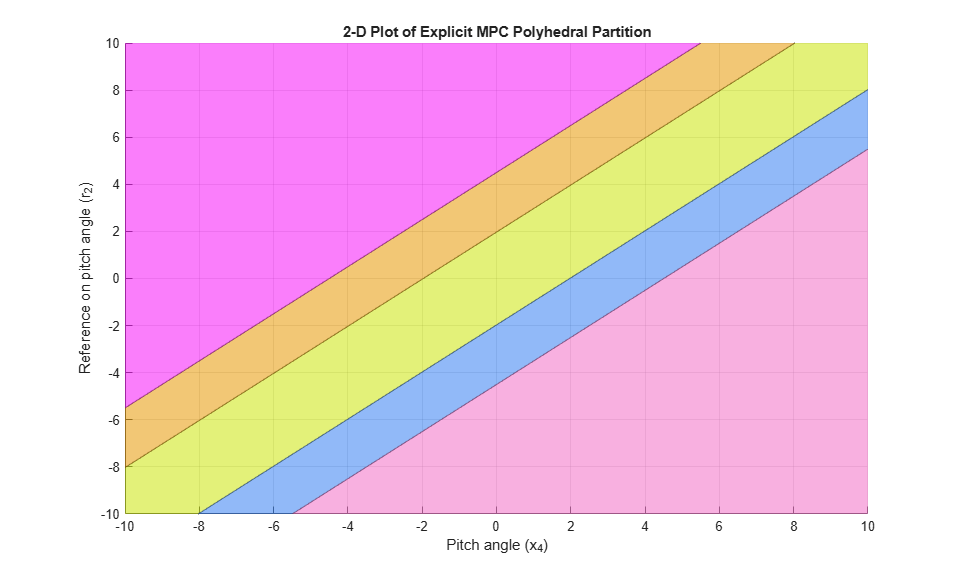Simulate closed-loop control of the linear plant model in Simulink. To do so, for the MPC Controller block, set the Explicit MPC Controller property to `empcobjSimplified`. For this example, this property is already set.

```mdl = 'empc_aircraft'; open_system(mdl) ```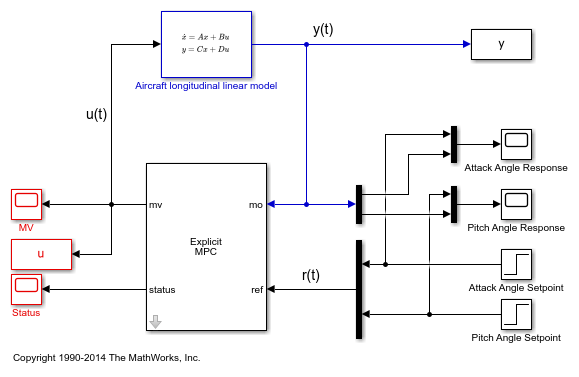Simulate the system from the command line using the Simulink `sim` command.

```sim(mdl) ```

Open the scopes showing the manipulated variables and the aircraft output response

```open_system('empc_aircraft/MV') open_system('empc_aircraft/Attack Angle Response') open_system('empc_aircraft/Pitch Angle Response') ```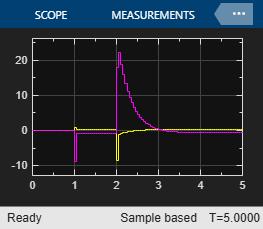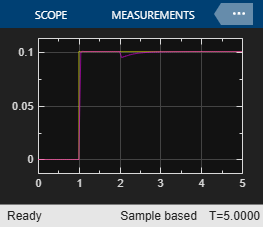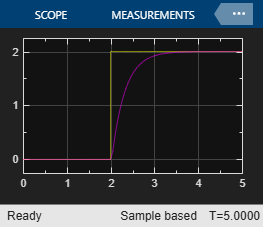The closed-loop response is identical to the one featured by the traditional MPC controller designed in MPC Control of an Aircraft with Unstable Poles.

### References

 P. Kapasouris, M. Athans, and G. Stein, "Design of feedback control systems for unstable plants with saturating actuators", Proc. IFAC Symp. on Nonlinear Control System Design, Pergamon Press, pp.302--307, 1990

 A. Bemporad, A. Casavola, and E. Mosca, "Nonlinear control of constrained linear systems via predictive reference management", IEEE® Trans. Automatic Control, vol. AC-42, no. 3, pp. 340-349, 1997.

```bdclose(mdl) ```